4727.0.55.002 - Australian Aboriginal and Torres Strait Islander Health Survey: Users' Guide, 2012-13
ARCHIVED ISSUE Released at 11:30 AM (CANBERRA TIME) 27/11/2013  First Issue
 Page tools: .mffeedback,#pjs { display :none; } Enable Javascript to Print PagesPrint All STANDARD ERRORS AND REPLICATE WEIGHTS Reliability of Estimates Sample Survey Errors 1 Two types of error are possible in estimates based on a sample survey: sampling error non-sampling error. 2 Sampling error occurs because only a small proportion of the total population is used to produce estimates that represent the whole population. Sampling error can be reliably measured, as it is calculated based on the scientific methods used to design surveys. 3 Non-sampling error may occur in any data collection, whether it is based on a sample or a full-count (i.e. Census). Non-sampling error may occur at any stage throughout the survey process. Examples include: non-response by selected persons questions being misunderstood responses being incorrectly recorded errors in coding or processing the survey data. 4 More detailed information on sample survey errors, including sampling error, non-sampling error and response rates is provided in Data Quality and Interpretation of Results. Sampling Error 5 Sampling error is the expected difference that could occur between the published estimates, derived from repeated random samples of persons, and the value that would have been produced if all persons in scope of the survey had been included. The magnitude of the sampling error associated with an estimate depends on the sample design, sample size and population variability. Measures of sampling error 6 A measure of the sampling error for a given estimate is provided by the Standard Error (SE), which is the extent to which an estimate might have varied by chance because only a sample of persons was obtained. 7 Another measure is the Relative Standard Error (RSE), which is the SE expressed as a percentage of the estimate. This measure provides an indication of the percentage errors likely to have occurred due to sampling. 8 Another measure is the Margin of Error (MoE), which describes the distance from the population value that the sample estimate is likely to be within, and is specified at a given level of confidence. Confidence levels typically used are 90%, 95% and 99%. For example, at the 95% confidence level the MoE indicates that there are about 19 chances in 20 that the estimate will differ by less than the specified MoE from the population value (the figure obtained if all dwellings had been enumerated). The 95% MoE is calculated as 1.96 multiplied by the SE. 9 The 95% MoE can also be calculated from the RSE by: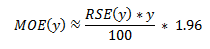10 The MoEs in this publication are calculated at the 95% confidence level. This can easily be converted to a 90% confidence level by multiplying the MoE by: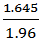or to a 99% confidence level by multiplying by a factor of: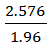11 A confidence interval expresses the sampling error as a range in which the population value is expected to lie at a given level of confidence. The confidence interval can easily be constructed from the MoE of the same level of confidence by taking the estimate plus or minus the MoE of the estimate. Examples of interpretation of sampling error 12 Standard errors can be calculated using the estimates and the corresponding RSEs. For example, in the 2011-12 AATSIHS First Results the estimated proportion of Aboriginal and Torres Strait Islander males aged 18 years and over who are current daily smokers is 42.9%. The RSE for this estimate is 3.5%, and the SE is calculated by: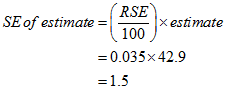13 Standard errors can also be calculated using the MoE. For example the MoE for the estimate of the proportion of Aboriginal and Torres Strait Islander males aged 18 years and over who are current daily smokers is +/- 2.9 percentage points. The SE is calculated by: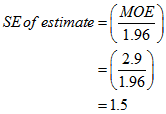14 Note due to rounding, the SE calculated from the RSE may be slightly different to the SE calculated from the MoE for the same estimate. 15 There are about 19 chances in 20 that the estimate of the proportion of Aboriginal and Torres Strait Islander males aged 18 years and over who are currently daily smokers is within +/- 2.9 percentage points from the population value. 16 Similarly, there are about 19 chances in 20 that the proportion of Aboriginal and Torres Strait Islander males aged 18 years and over who are currently daily smokers is within the confidence interval of 40.0% to 45.8%. Standard errors of derived estimates of proportions 17 Proportions formed from the ratio of two estimates are also subject to sampling errors. The size of the error depends on the accuracy of both the numerator and denominator. For proportions where the denominator is an estimate of the number of persons in a group, and the numerator is the number of persons in a sub-group of the denominator population, a formula to approximate the RSE is: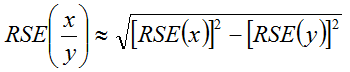For example, the proportion of those with cardiovascular disease (denominator) who have seen a doctor (numerator). 18 Using this formula, the RSE of the estimated proportion will be lower than the RSE estimate of the numerator. Therefore another approximation for SEs of proportions may be derived by neglecting the RSE of the denominator; i.e. obtaining the RSE of the number of persons corresponding to the numerator of the proportion and then applying this figure to the estimated proportion. Standard error of a difference 19 The difference between two survey estimates is itself an estimate, and is therefore subject to sampling variability. The sampling error of the difference between the two estimates depends on their individual SEs and the level of statistical association (correlation) between the estimates. An approximate SE of the difference between two estimates (x-y) may be calculated by the following formula: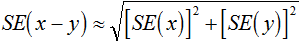For example, the number of male smokers minus the number of female smokers. 20 While this formula will only be exact for differences between separate sub-populations or uncorrelated characteristics of sub-populations, it is expected to provide a reasonable approximation for most differences likely to be of interest in relation to this survey. Standard error of a sum 21 The sum of two survey estimates is itself an estimate and is therefore subject to sampling variability. The sampling error of the sum of the two estimates depends on their individual SEs and the level of statistical association (correlation) between the estimates. An approximate SE of the sum of two estimates (x+y) may be calculated by the following formula: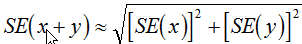22 For example the number of people with asthma plus the number of people with hayfever. 23 While this formula will only be exact for sums of separate sub-populations or uncorrelated characteristics of sub-populations, it is expected to provide a reasonable approximation for most estimates likely to be of interest in relation to this survey. Relative standard error and Margin of Error for derived proportions, differences and sums 24 The approximate RSE for differences and sums can be calculated from the SE by: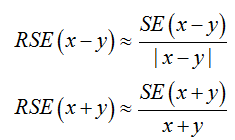25 The approximate 95% MoE for proportions, differences and sums can be calculated by: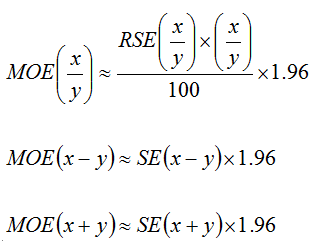Replicate Weights Technique 26 A class of techniques called 'replication methods' provide a general method of estimating variances for the types of complex sample designs and weighting procedures employed in ABS household surveys. 27 The basic idea behind the replication approach is to select sub-samples repeatedly from the whole sample, for each of which the statistic of interest is calculated. The variance of the full sample statistic is then estimated using the variability among the replicate statistics calculated from these sub-samples. The sub-samples are called 'replicate groups', and the statistics calculated from these replicates are called 'replicate estimates'. 28 There are various ways of creating replicate sub-samples from the full sample. The replicate weights produced for the 2012-13 AATSIHS were created under the delete-a-group Jackknife method of replication (described below). 29 There are numerous advantages to using the replicate weighting approach, including the fact that: the same procedure is applicable to most statistics such as means, percentages, ratios, correlations, derived statistics and regression coefficients; and it is not necessary for the analyst to have available detailed survey design information if the replicate weights are included with the data file. Derivation of replicate weights 30 Under the delete-a-group Jackknife method of replicate weighting, weights were derived as follows: 250 replicate groups were formed, with each group formed to mirror the overall sample. Units from a cluster of dwellings all belong to the same replicate group, and a unit can belong to only one replicate group. For each replicate weight, one replicate group was omitted from the weighting and the remaining records were weighted in the same manner as for the full sample. The records in the group that was omitted received a weight of zero. This process was repeated for each replicate group (i.e. a total of 250 times). Ultimately each record had 250 replicate weights attached to it with one of these being the zero weight. Application of replicate weights 31 As noted above, replicate weights enable variances of estimates to be calculated relatively simply. They also enable unit record analyses such as chi-square and logistic regression to be conducted, which take into account the sample design. 32 Replicate weights for any variable of interest can be calculated from the 250 replicate groups, giving 250 replicate estimates. The distribution of this set of replicate estimates, in conjunction with the full sample estimate, is then used to approximate the variance of the full sample. 33 The formulae for calculating the standard error (SE), relative standard error (RSE) and 95% Margin of Error (MoE) of an estimate using this method are shown below: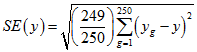34 where: g = (1, ..., 250) (the number of replicate weights) y(g) = estimate from using replicate weighting y = estimate from using full person weight. 35 The RSE(y) = SE(y)/y*100. 36 The 95% MoE(y)=SE(y)*1.96. 37 This method can also be used when modelling relationships from unit record data, regardless of the modelling technique used. In modelling, the full sample would be used to estimate the parameter being studied (such as a regression coefficient); i.e. the 250 replicate groups would be used to provide 250 replicate estimates of the survey parameter. The variance of the estimate of the parameter from the full sample is then approximated, as above, by the variability of the replicate estimates. Availability of RSEs calculated using replicate weights 38 Actual RSEs for all estimates and proportion estimates have been calculated in the publications released for the AATSIHS. The RSEs for estimates are available in spreadsheet format (datacubes) accessed by clicking on the downloads tab of the AATSIHS products. The RSEs in the spreadsheets were calculated using the replicate weights methodology. Availability of MoEs calculated using replicate weights 39 Actual MoEs for proportion estimates have been calculated in the publications released for the AATSIHS. The MoEs for estimates published in the AATSIHS are available in spreadsheet format (datacubes) accessed by clicking on the downloads tab. The MoEs in the spreadsheets were calculated using the replicate weights methodology. Document Selection These documents will be presented in a new window.